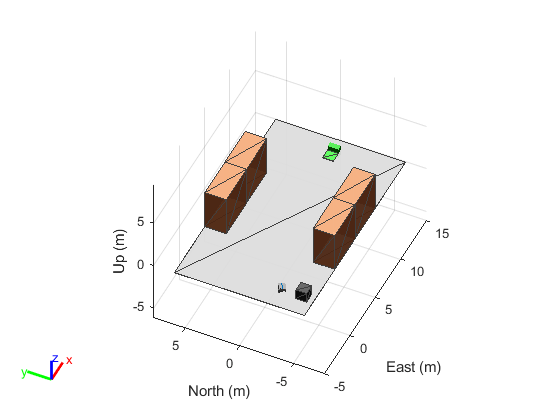Package: robotics

Custom robot sensor interface

Since R2022b

## Description

The `robotics.SensorAdaptor` class is an interface for adapting custom sensor models to for use with the `robotScenario` object for robot scenario simulation.

The `robotics.SensorAdaptor` class is a `handle` class.

### Class Attributes

 `Abstract` `true`

For information on class attributes, see Class Attributes.

## Creation

### Syntax

``sensorObj = robotics.SensorAdaptor(sensorModel)``

### Description

example

````sensorObj = robotics.SensorAdaptor(sensorModel)` creates a sensor object compatible with the `robotScenario` object. `sensorModel` is an object handle for a custom implementation of the `SensorAdaptor` class.To get a template for a custom sensor implementation, use the `createCustomRobotSensorTemplate` function.```

## Properties

expand all

Sensor update rate, specified as a positive scalar in Hz.

Example: `10`

Data Types: `double`

Custom sensor model implementation, specified as an object handle. To get a template for a custom sensor implementation, use the `createCustomRobotSensorTemplate` function.

#### Attributes:

 `SetAccess` `private`

expand all

## Examples

collapse all

This example focuses on creating and mounting an ultrasonic sensor on a mobile robot in a `robotScenario`. The `ultrasonicDetectionGenerator` from the Automated Driving Toolbox cannot be used directly with `robotScenario`. We will be implementing a custom sensor adaptor for the `ultrasonicDetectionGenerator` that makes it compatible with `robotScenario`. The sensor will be used to position a mobile robot correctly at a charging station.

Use the `createCustomRobotSensorTemplate` function to generate a template sensor and update it to adapt an `ultrasonicDetectionGenerator` object for usage in Robot scenario.

```createCustomRobotSensorTemplate ```

This example provides the adaptor class `CustomUltrasonicSensor`, which can be viewed using the following command.

```edit CustomUltrasonicSensor.m ```

Use the Sensor Adaptor in Robot Scenario Simulation

Create a `robotScenario` object with a sample rate of 10.

```sampleRate = 10; scenario = robotScenario(UpdateRate=sampleRate); ```

Add a plane mesh to show the warehouse floor.

`addMesh(scenario,"Plane",Position=[5 0 0],Size=[20 12],Color=[0.7 0.7 0.7]);`

Create a `waypointTrajectory` that traverses a set of waypoints to the charging station and use the `lookupPose` method of the `waypointTrajectory` object to fetch the pose of the robot along the trajectory.

```startPosition = [-3 -3]; chargingPosition = [13 0]; wPts = [[startPosition 0.1]; ... 5 0 0.1; ... 10 0 0.1; ... 13.75 0 0.1]; %Charging station toa = [0 4 7 10]; traj = waypointTrajectory(Waypoints=wPts,... TimeOfArrival=toa, ReferenceFrame='ENU', ... SampleRate=sampleRate); [pos, orient, vel, acc, angvel] = traj.lookupPose(0:1/sampleRate:10);```

Add a `robotPlatform` to the scene for our mobile robot. Load the Clearpath Husky model for the `rigidBodyTree` of the `robotPlatform`. Also add cuboid meshes to denote obstacles in the scene. Add a 1-by-1 plane to denote where the charging station is.

```robot = robotPlatform("rst", scenario,... RigidBodyTree=loadrobot("clearpathHusky"), ... InitialBasePosition=pos(1,:), InitialBaseOrientation=compact(orient(1))); addMesh(scenario,"Box",Position=[3 5 2],Size=[4 2 4],Color=[1 0.5 0.25],IsBinaryOccupied=true); addMesh(scenario,"Box",Position=[3 -5 2],Size=[4 2 4],Color=[1 0.5 0.25],IsBinaryOccupied=true); addMesh(scenario,"Box",Position=[7 5 2],Size=[4 2 4],Color=[1 0.5 0.25],IsBinaryOccupied=true); addMesh(scenario,"Box",Position=[7 -5 2],Size=[4 2 4],Color=[1 0.5 0.25],IsBinaryOccupied=true); addMesh(scenario,"Box",Position=[-3 -5 0.5],Size=[1 1 1],Color=[0.1 0.1 0.1]); % Plane to denote Charging station location addMesh(scenario,"Plane",Position=[13 0 .05],Size=[1 1],Color=[0 1 0]);```

Create the charging station using a `robotPlatform` object. The `robotPlatform` allows us to fetch the transform between the object and the sensor for use in the custom sensor read method. Here, the charging station can be modeled using a cuboid. The robot has to reach within 5cm of the surface of the charging station to start charging.

```chargeStation = robotPlatform("chargeStation", scenario,InitialBasePosition=[13.75 0 0]); chargeStation.updateMesh("Cuboid",Size=[0.5 1 1], Color=[0 0.8 0]);```

The ultrasonic sensor model requires inputs of the profile of the obstacles to be detected. The profile structure includes information about the dimensions of the obstacle.

`chargingStationProfile = struct("Length", 0.5, "Width", 1, "Height", 1, 'OriginOffset', [0 0 0]);`

Create the ultrasonic sensor using the `ultrasonicDetectionGenerator` object and set its mounting location to `[0 0 0]`, detection range to `[0.03 0.04 5]` and field of view to `[70, 35]`. Also pass in the profile of the charging station that was created earlier.

```ultraSonicSensorModel = ultrasonicDetectionGenerator(MountingLocation=[0 0 0], ... DetectionRange=[0.03 0.04 5], ... FieldOfView=[70, 35], ... Profiles=chargingStationProfile);```

Create a `robotSensor` object that uses the custom sensor adaptor `CustomUltrasonicSensor`. The adaptor uses the ultrasonic sensor model created above. The mounting location will be at the front of the robot.

```ult = robotSensor("UltraSonic", robot, ... CustomUltrasonicSensor(ultraSonicSensorModel), ... MountingLocation=[0.5 0 0.05]); figure(1); ax = show3D(scenario); view(-65,45) light grid on```In this scene, the mobile robot will follow the trajectory to the charging station. When the ultrasonic sensor comes within a range of 20cm of the charging station, then mobile robot advance at a slower rate of 1cm per frame towards the charging station. When the robot is within 5cm of the surface of the charging station, it stops and the charging starts. The simulation ends when the charging starts.

```isCharging = false; i = 1; setup(scenario); while ~isCharging [isUpdated, t, det, isValid] = read(ult); figure(1); show3D(scenario); view(-65,45) light grid on % Read the motion vector of the robot from the platform ground truth % This motion vector will be used only for plotting graphic elements pose = robot.read(); rotAngle = quat2eul(pose(10:13)); hold on if ~isempty(det) % Distance to object distance = det{1}.Measurement; % Plot a red shpere where the ultrasonic sensor detects an object exampleHelperPlotDetectionPoint(scenario, ... det{1}.ObjectAttributes{1}.PointOnTarget, ... ult.Name, ... pose); displayText = ['Distance = ',num2str(distance)]; else distance = inf; displayText = 'No object detected!'; end % Plot a cone to represent the field of view and range of the ultrasonic sensor exampleHelperPlotFOVCylinder(pose, ultraSonicSensorModel.DetectionRange(3)); hold off if distance <= 0.2 % Advance in steps of 1cm when the robot is within 20cm of the charging station currentMotion = lastMotion; currentMotion(1) = currentMotion(1) + 0.01; move(robot,"base",currentMotion); lastMotion = currentMotion; displayText = ['Detected Charger! Distance = ',num2str(distance)]; if distance <= 0.05 % The robot is charging when it is within 5cm of the charging station displayText = ['Charging!! Distance = ',num2str(distance)]; isCharging = true; end else % Follow the waypointTrajectory to the vicinity of the charging station if i<=length(pos) motion = [pos(i,:), vel(i,:), acc(i,:), ... compact(orient(i)), angvel(i,:)]; move(robot,"base",motion); lastMotion = motion; i=i+1; end end % Display the distance to the charging station detected by the ultrasonic sensor t = text(15, 0, displayText, "BackgroundColor",'yellow'); t(1).Color = 'black'; t(1).FontSize = 10; advance(scenario); updateSensors(scenario); end```## Version History

Introduced in R2022b